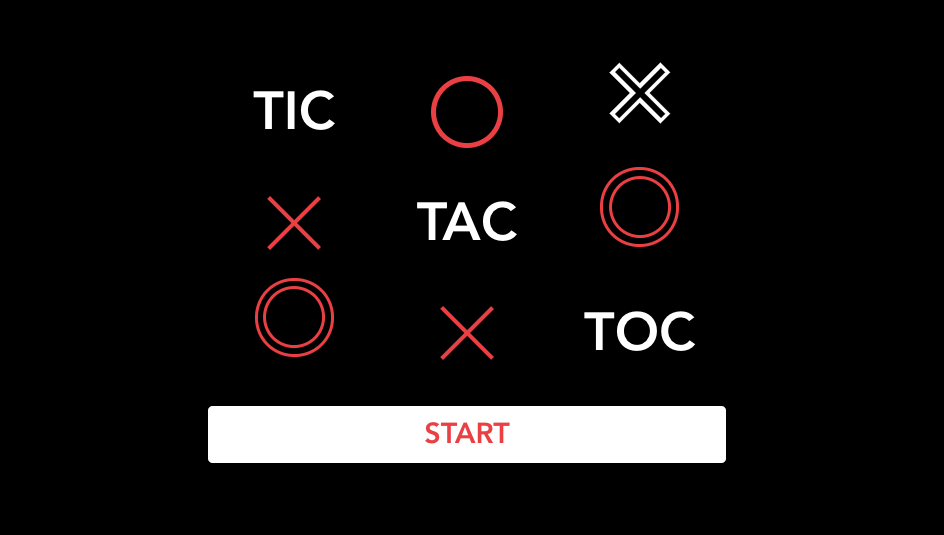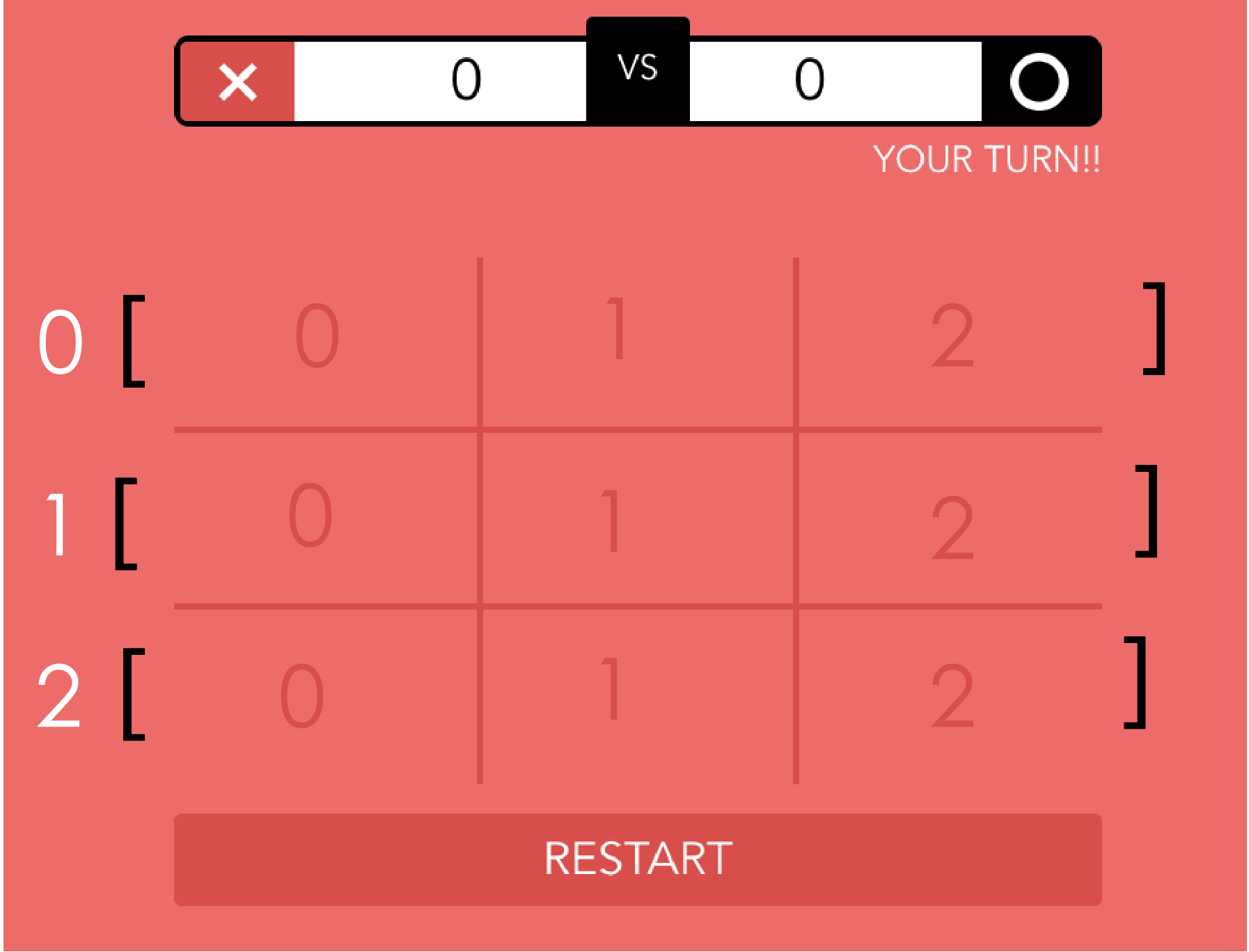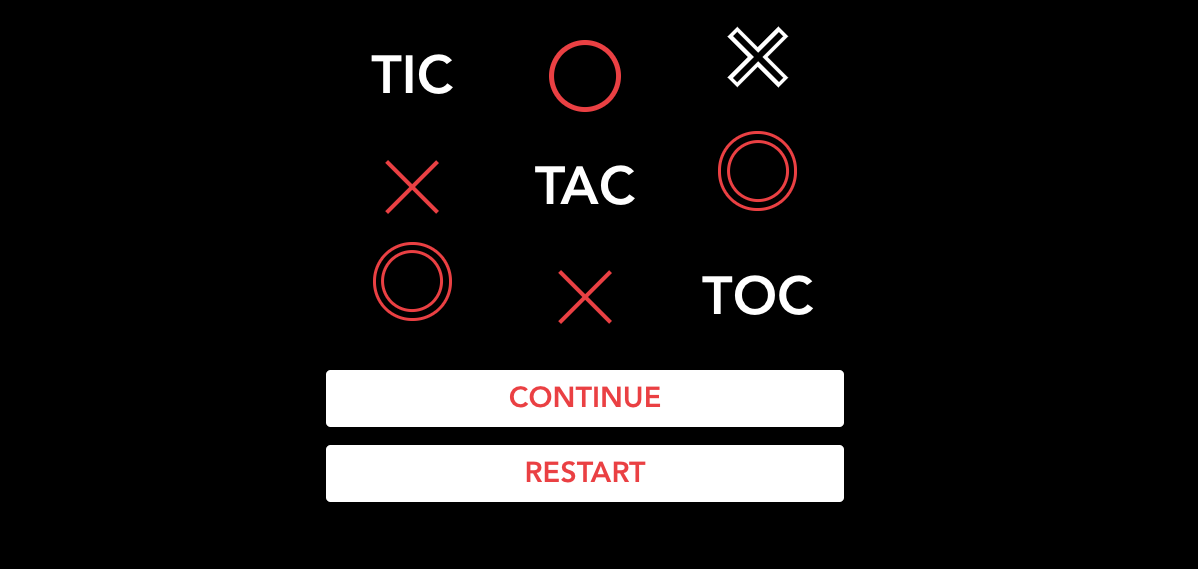# Vue.js 井字遊戲 - OOXX## 規則

• 先手為 Ｏ，後手為 X，某方獲勝時，上方會紀錄各方的獲勝戰績

• 每回合結束後，會判定結果頁(平手、Ｏ獲勝、X獲勝)

• 需符合 RWD，能在低螢幕解析度也能遊玩，介面不能超出 x 軸，至少在以下解析度能夠遊玩:

1. iPhone SE 320px
2. iPhone 8 375px
3. iPhone PLUS 414px
• 請使用瀏覽器離線儲存技術，將戰績保留起來

## 資料結構

data(){
return {
...
currentPlayer:0,
gameStatus:[[null,null,null],
[null,null,null],
[null,null,null]]
}}## 判斷勝利邏輯

• 每個Row（橫排）是否有連線
• 每個Column（直行）是否有連線
• 對角線是否有連線

methods:{
...
onSectionClick(x,y){
if (this.gameStatus[y][x]!==null) return //防止重複下棋
this.gameStatus[y][x] = this.currentPlayer//下棋
this.checkResult()//檢查賽況
this.togglePlayer()//<---下完後切換使用者

},}


  let status = this.gameStatus
for(let y=0; y<status.length; y++){
for(let x=0; x<status[y].length; x++ ){
// 用status[y][x]取得棋格元素 <-----重要
}
}



### 檢查Row / Column是否有連線

methods:{
...
getSumOfRow(x,y){
let status = this.gameStatus
if(x!==0 ) return
return status[y][x]+
status[y][x+1]+
status[y][x+2]
},}


Coloum總和則為x y與上述相反：

 getSumOfColumn(x,y){
let status = this.gameStatus
if(y!==0 ) return
return   status[y][x]+
status[y+1][x]+
status[y+2][x]
},


### 檢查對角線是否有連線

methods:{
getSumOfNegativeSlash(x,y){
let status = this.gameStatus
if(x!==0 || y!==0 ) return
if(  !(this.isNumber(status[y][x]) &&
this.isNumber(status[y+1][x+1]) &&
this.isNumber(status[y+2][x+2]))) return

return   status[y][x]+ status[y+1][x+1]+status[y+2][x+2]
},

}


### 整合所有的判斷邏輯 - checkResult method

         checkResult(){

let status = this.gameStatus
for(let y=0; y<status.length; y++){
for(let x=0; x<status[y].length; x++ ){
let sumOfCol = this.getSumOfColumn(x,y)
let sumOfRow = this.getSumOfRow(x,y)
if(
(   this.isRowAllNumber(x,y) &&
sumOfRow=== 0||sumOfRow=== 3
)||(
this.isColumnAllNumber(x,y) &&
sumOfCol === 0||sumOfCol === 3
)||(
this.getSumOfNegativeSlash(x,y)===0 ||
this.getSumOfNegativeSlash(x,y)===3 ||
this.getSumOfPositiveSlash(x,y)===0 ||
this.getSumOfPositiveSlash(x,y)===3
)){
this.setScore()
this.goResultPage(this.currentPlayer)
}
}
}
this.checkIfFinish()
},


## 使用localStorage紀錄分數

• localStorage.setItem(‘key’,‘content’)
• localStorage.getItem(‘key’)
• localStorage.removeItem(‘key’)

export const setStorage = (name, content) => {
if (!name) return;
if (typeof content !== "string") {
content = JSON.stringify(content);
}
window.localStorage.setItem(name, content);
};


getStorage、removeStorage則是類似的方式，在此就不多提。

## 多思考一些 - 重置分數按鈕